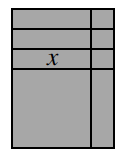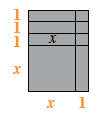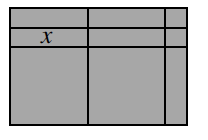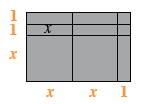### Home > INT2 > Chapter 1 > Lesson 1.3.4 > Problem1-114

1-114.

Examine the rectangles formed with tiles below. For each figure, write an equation showing that its area as a product of the width and length is equal to its area as a sum of its parts.

1.Find the outside dimensions of the large rectangle.

See dimensions below.As a product: multiply the dimensions of the large rectangle together.
As a sum: add up the areas of all of the small rectangles.

$\text{area as product} = \text{(length)(width)} = (x + 3)(x + 1)$
$\text{area as sum} = x + x + x + x^2 + 1 + 1 + 1 + x = x^2 + 4x + 3$

1.Use the same method as part (a).$\text{area as a product} = \\ \text{(length)(width)} = (2x + 1)(x + 2)$
$\text{product as a sum} = \\ x^2 + x^2 + x+ x + x + x + x + 1 + 1 = 2x^2 + 5x + 2$